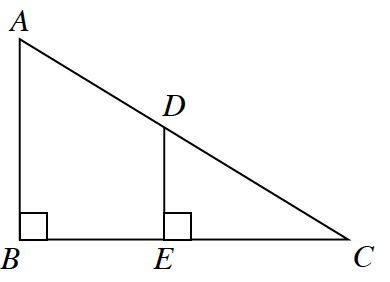### Home > GC > Chapter 4 > Lesson 4.2.4 > Problem4-83

4-83.Are the triangles at right similar? If so, write a flowchart that justifies your conclusion. If not, explain how you know.

Draw triangles $ABC$ and $DEC$ separately. What do you notice about angles $B$ and $E$? What do you notice about angle $C$?